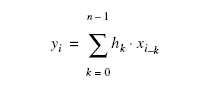# FIR Filters

NI High-Speed Digitizers Help (NI-SCOPE)

Edition Date: January 2017

Part Number: 370592AB-01

View Product InfoNI-SCOPE (English | Japanese)

Finite impulse response (FIR) filters are digital filters that have a finite impulse response. FIR filters are also known as nonrecursive filters, convolution filters, or moving-average (MA) filters because you can express the output of a FIR filter as a finite convolution:where x represents the input sequence to be filtered, y represents the output filtered sequence, and h represents the FIR filter coefficients.

The most important characteristics of FIR filters are the following:

• They can achieve linear phase because of filter coefficient symmetry in the realization.
• They are always stable.
• The filtering function is performed using the convolution and, as such, generally allows associating a delay with the output sequence of (n-1)/2, where n is the number of filter coefficients or filter taps.

The simplest method for designing linear-phase FIR filters is the window design method. To design a FIR filter by windowing, you start with an ideal frequency response, calculate its impulse response, and then truncate the impulse response to produce a finite number of coefficients. The truncation of the ideal impulse response results in the effect known as the Gibbs phenomenon—oscillatory behavior near abrupt transitions (cutoff frequencies) in the FIR filter frequency response.

You can reduce the effects of the Gibbs phenomenon by smoothing the truncation of the ideal impulse response using a smoothing window. By tapering the FIR coefficients at each end, you can diminish the height of the side lobes in the frequency response. This method widens the main lobe, however, resulting in a wider transition region at the cutoff frequencies. The selection of a window function, then, is similar to the choice between Chebyshev and Butterworth IIR filters in that it is a trade-off between side lobe levels near the cutoff frequencies and width of the transition region.

### Related Topics

Types of FIR Filters in NI-SCOPE

IIR Filters

Digital Filtering